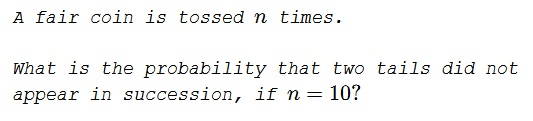# Probability of No Two-Tail Runs

### Problem### Solution 1

Let $X_n$ be the number of $H/T$ strings with no two successive $T.$ $X_n$ = $T_n$+$H_n$ to distinguish the strings that end in $T$ from those that end with $H.$

Obviously, $H_1=T_1=1,\,H_n=H_{n-1}+T_{n-1},$ and $T_n=H_{n-1}.$ Thus me may compute

$\begin{array}{c|c|c|c|c|c|c|c|c|c|c} n&1&2&3&4&5&6&7&8&9&10\\ \hline T_n&1&2&3&5&8&13&21&34&55&89\\ H_n&1&1&2&3&5&8&13&21&34&55 \end{array}$

It follows that there are $X_{10}=T_{10}+H_{10}=89+55=144$ ten letters long strings with no $TT.$ There are $2^{10}=1024$ ten letter strings in all. Thus the probability of not seeing $TT$ is $\displaystyle\frac{144}{1024}=\frac{9}{64}.$

### Solution 2

Let $\bf{M}$ be a Markov chain:

$\bf{M}=\left( \begin{array}{ccc} \frac{1}{2} & \frac{1}{2} & 0 \\ \frac{1}{2} & 0 & \frac{1}{2} \\ 0 & 0 & 1 \\ \end{array} \right)$

representing transitions between the following states:

$\begin{array}{c|c|c|c} & \text{N} & \text{T} & \text{TT} \\ \hline \text{N} & \frac{1}{2} & \frac{1}{2} & 0 \\ \hline \text{T} & \frac{1}{2} & 0 & \frac{1}{2} \\ \hline \text{TT} & 0 & 0 & 1 \\ \end{array}$

The matrix at the $10^{th}$ step is $\bf{M}^{10}$ and the probabilities are

$(1,0,0).\bf{M}^{10}=\left( \begin{array}{c} p(N)_{10} \\ p(T)_{10} \\ p(TT)_{10} \\ \end{array} \right) =\left( \begin{array}{c} \frac{89}{1024} \\ \frac{55}{1024} \\ \frac{55}{64} \\ \end{array} \right)$

So the probability of never getting two tails in ten throws is

$\displaystyle 1-p(TT)_{10}=1-\frac{55}{64}=\frac{9}{64}.$

### Acknowledgment

This is problem P1988-7 from A Friendly Mathematics Competition by Rick Gillman (ed.)

Solution 2 is by N. N. Taleb.Copyright © 1996-2018 Alexander Bogomolny

 64859747